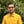Trusted answers to developer questions
Trusted Answers to Developer Questions

Related Tags

scala
math
communitycreator

# What is Math.max() in Scala?Harris Amjad

Grokking Modern System Design Interview for Engineers & Managers

Ace your System Design Interview and take your career to the next level. Learn to handle the design of applications like Netflix, Quora, Facebook, Uber, and many more in a 45-min interview. Learn the RESHADED framework for architecting web-scale applications by determining requirements, constraints, and assumptions before diving into a step-by-step design process.

The max() function returns the largest value out of the two numbers sent as a parameter. Figure 1 shows a visual representation of the max() function.

Figure 1: Visual representation of max() function

The following module is required for this function:

import scala.math._


### Syntax

type max(type num-1, type num-2)
// Type can be : Int, Float, Double, Long


### Parameter

The max() function takes two numbers as a parameter.

The numbers can be of type Int, Double, Float, or Long.

### Return value

The max() function returns the largest value from the two numbers sent as a parameter.

• If either of the two parameter values is NaN, or if both are NaN, then it returns NaN.

### Code

import scala.math._object Main extends App {    // two positive numbers    println(s"The value of max(10, 0) = ${max(10, 0)}"); //one positive and the other negative println(s"The value of max(4, -6) =${max(4, -6)}");     println(s"The value of max(Double.PositiveInfinity,Double.PositiveInfinity) = ${max(Double.PositiveInfinity,Double.NegativeInfinity)}"); // both negative numbers println(s"The value of max(-10, -9) =${max(-10, -9)}");     // both double numbers    println(s"The value of max(12.234,1.2345) = ${max(12.234,1.2345)}"); // one int and the other double println(s"The value of max(12.234,1) =${max(12.234,1)}");    //error ouput    println(s"The value of max(Double.NaN,Double.NaN) = \${max(Double.NaN,Double.NaN)}"); }

RELATED TAGS

scala
math
communitycreator

CONTRIBUTORHarris Amjad

Grokking Modern System Design Interview for Engineers & Managers

Ace your System Design Interview and take your career to the next level. Learn to handle the design of applications like Netflix, Quora, Facebook, Uber, and many more in a 45-min interview. Learn the RESHADED framework for architecting web-scale applications by determining requirements, constraints, and assumptions before diving into a step-by-step design process.

Keep Exploring

Learn in-demand tech skills in half the time